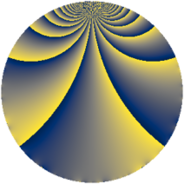# Properties

 Label 637.2.gLevel $637$ Weight $2$ Character orbit 637.g Rep. character $\chi_{637}(263,\cdot)$ Character field $\Q(\zeta_{3})$ Dimension $86$ Newform subspaces $13$ Sturm bound $130$ Trace bound $5$

# Related objects

## Defining parameters

 Level: $$N$$ $$=$$ $$637 = 7^{2} \cdot 13$$ Weight: $$k$$ $$=$$ $$2$$ Character orbit: $$[\chi]$$ $$=$$ 637.g (of order $$3$$ and degree $$2$$) Character conductor: $$\operatorname{cond}(\chi)$$ $$=$$ $$91$$ Character field: $$\Q(\zeta_{3})$$ Newform subspaces: $$13$$ Sturm bound: $$130$$ Trace bound: $$5$$ Distinguishing $$T_p$$: $$2$$, $$3$$, $$5$$

## Dimensions

The following table gives the dimensions of various subspaces of $$M_{2}(637, [\chi])$$.

Total New Old
Modular forms 146 102 44
Cusp forms 114 86 28
Eisenstein series 32 16 16

## Trace form

 $$86q - q^{2} + 8q^{3} - 41q^{4} + 2q^{5} + 6q^{6} - 12q^{8} + 66q^{9} + O(q^{10})$$ $$86q - q^{2} + 8q^{3} - 41q^{4} + 2q^{5} + 6q^{6} - 12q^{8} + 66q^{9} + 2q^{10} + 22q^{11} - 2q^{12} + 4q^{13} - 19q^{15} - 41q^{16} - 7q^{17} - 5q^{18} - 2q^{20} - 4q^{22} + 3q^{23} - 40q^{24} - 31q^{25} + 26q^{27} - 2q^{29} - 72q^{30} - 13q^{31} + 21q^{32} + 14q^{33} - 28q^{34} - 3q^{36} + q^{37} + 16q^{38} + 41q^{39} - 4q^{40} + 11q^{41} - 14q^{43} - 58q^{44} + 25q^{45} + 12q^{46} + 2q^{47} - 18q^{48} - 24q^{50} + 16q^{51} + 32q^{52} - 17q^{53} + 9q^{54} - 18q^{55} - 68q^{57} + 2q^{58} - 17q^{59} + 33q^{60} + 16q^{61} - 2q^{62} - 16q^{64} - 32q^{65} - 9q^{66} - 88q^{67} - 27q^{68} - 23q^{69} + q^{71} + 94q^{72} + 17q^{73} + 23q^{74} - 9q^{75} + 10q^{76} + 5q^{78} + 22q^{79} - 8q^{80} - 82q^{81} - 4q^{82} + 54q^{83} + 39q^{85} + 8q^{86} - 37q^{87} - 18q^{88} + 2q^{89} - 20q^{90} - 114q^{92} + 16q^{93} + 88q^{94} - 29q^{95} - 34q^{96} + 30q^{97} + 36q^{99} + O(q^{100})$$

## Decomposition of $$S_{2}^{\mathrm{new}}(637, [\chi])$$ into newform subspaces

Label Dim. $$A$$ Field CM Traces $q$-expansion
$$a_2$$ $$a_3$$ $$a_5$$ $$a_7$$
637.2.g.a $$2$$ $$5.086$$ $$\Q(\sqrt{-3})$$ None $$-1$$ $$6$$ $$3$$ $$0$$ $$q-\zeta_{6}q^{2}+3q^{3}+(1-\zeta_{6})q^{4}+(3-3\zeta_{6})q^{5}+\cdots$$
637.2.g.b $$4$$ $$5.086$$ $$\Q(\sqrt{-3}, \sqrt{5})$$ None $$-3$$ $$-6$$ $$-3$$ $$0$$ $$q+(-1-\beta _{1}-\beta _{3})q^{2}+(-1+\beta _{2})q^{3}+\cdots$$
637.2.g.c $$4$$ $$5.086$$ $$\Q(\sqrt{-3}, \sqrt{5})$$ None $$-3$$ $$6$$ $$3$$ $$0$$ $$q+(-1-\beta _{1}-\beta _{3})q^{2}+(1-\beta _{2})q^{3}+\cdots$$
637.2.g.d $$4$$ $$5.086$$ $$\Q(\zeta_{12})$$ None $$0$$ $$-4$$ $$0$$ $$0$$ $$q-\zeta_{12}^{2}q^{2}+(-1-\zeta_{12}^{3})q^{3}+(-1+\cdots)q^{4}+\cdots$$
637.2.g.e $$4$$ $$5.086$$ $$\Q(\zeta_{12})$$ None $$0$$ $$4$$ $$0$$ $$0$$ $$q-\zeta_{12}^{2}q^{2}+(1+\zeta_{12}^{3})q^{3}+(-1+\zeta_{12}+\cdots)q^{4}+\cdots$$
637.2.g.f $$4$$ $$5.086$$ $$\Q(\sqrt{2}, \sqrt{-3})$$ None $$2$$ $$0$$ $$-2$$ $$0$$ $$q+(1+\beta _{1}+\beta _{2})q^{2}+\beta _{3}q^{3}+(2\beta _{1}+\beta _{2}+\cdots)q^{4}+\cdots$$
637.2.g.g $$4$$ $$5.086$$ $$\Q(\sqrt{2}, \sqrt{-3})$$ None $$2$$ $$0$$ $$2$$ $$0$$ $$q+(1+\beta _{1}+\beta _{2})q^{2}-\beta _{3}q^{3}+(2\beta _{1}+\beta _{2}+\cdots)q^{4}+\cdots$$
637.2.g.h $$8$$ $$5.086$$ 8.0.$$\cdots$$.7 None $$-4$$ $$0$$ $$0$$ $$0$$ $$q+(-1+\beta _{2})q^{2}+(-\beta _{5}+\beta _{6})q^{3}+\beta _{2}q^{4}+\cdots$$
637.2.g.i $$8$$ $$5.086$$ 8.0.$$\cdots$$.6 None $$-2$$ $$0$$ $$0$$ $$0$$ $$q+(\beta _{2}+\beta _{5})q^{2}+\beta _{3}q^{3}+(-2-2\beta _{2}+\cdots)q^{4}+\cdots$$
637.2.g.j $$8$$ $$5.086$$ $$\mathbb{Q}[x]/(x^{8} - \cdots)$$ None $$1$$ $$-2$$ $$-7$$ $$0$$ $$q+\beta _{1}q^{2}-\beta _{6}q^{3}+(-1+\beta _{1}+\beta _{2}+\cdots)q^{4}+\cdots$$
637.2.g.k $$8$$ $$5.086$$ $$\mathbb{Q}[x]/(x^{8} - \cdots)$$ None $$1$$ $$2$$ $$7$$ $$0$$ $$q+\beta _{1}q^{2}+\beta _{6}q^{3}+(-1+\beta _{1}+\beta _{2}+\cdots)q^{4}+\cdots$$
637.2.g.l $$12$$ $$5.086$$ $$\mathbb{Q}[x]/(x^{12} - \cdots)$$ None $$2$$ $$2$$ $$-1$$ $$0$$ $$q+(-\beta _{1}-\beta _{5}+\beta _{11})q^{2}+(-\beta _{3}+\beta _{11})q^{3}+\cdots$$
637.2.g.m $$16$$ $$5.086$$ $$\mathbb{Q}[x]/(x^{16} + \cdots)$$ None $$4$$ $$0$$ $$0$$ $$0$$ $$q+(1+\beta _{4}+\beta _{10})q^{2}+(\beta _{1}-\beta _{5}+\beta _{8}+\cdots)q^{3}+\cdots$$

## Decomposition of $$S_{2}^{\mathrm{old}}(637, [\chi])$$ into lower level spaces

$$S_{2}^{\mathrm{old}}(637, [\chi]) \cong$$ $$S_{2}^{\mathrm{new}}(91, [\chi])$$$$^{\oplus 2}$$